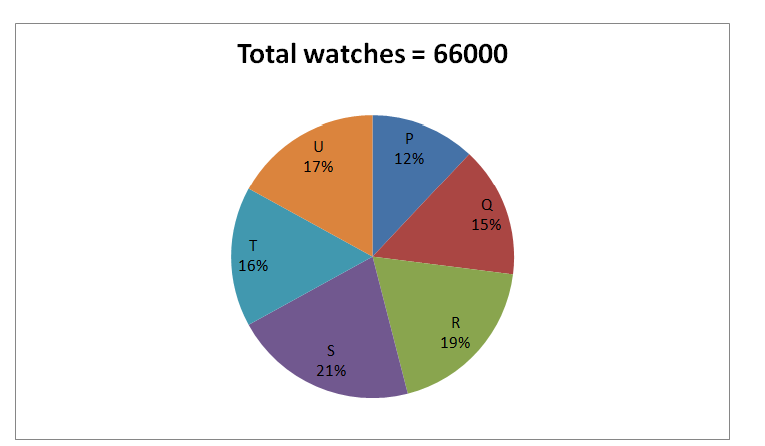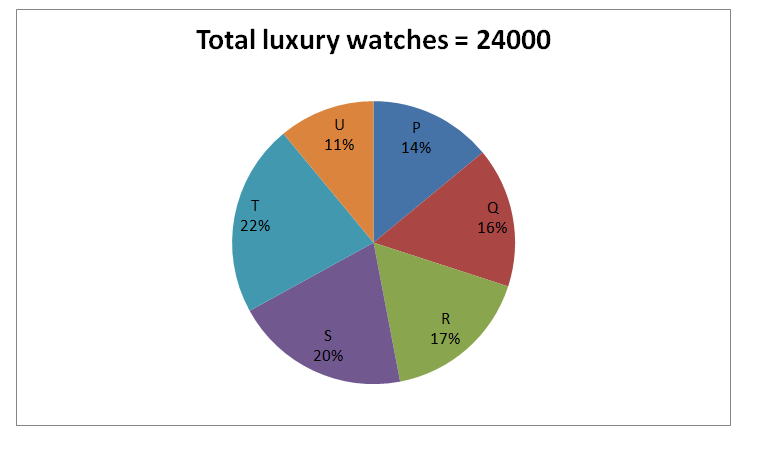# SBI PO Prelims Quantitative Aptitude Questions 2019 (Day-54)

Dear Aspirants, Our IBPS Guide team is providing new series of Quantitative Aptitude Questions for SBI PO 2019 so the aspirants can practice it on a daily basis. These questions are framed by our skilled experts after understanding your needs thoroughly. Aspirants can practice these new series questions daily to familiarize with the exact exam pattern and make your preparation effective.

[WpProQuiz 6429]

### Click Here for SBI PO Pre 2019 High-Quality Mocks Exactly on SBI Standard

1) A and B enter into a partnership by investing Rs. 30000 and Rs. 40000 respectively. After 4 months, C enters into a partnership by investing Rs. 50000. Find the share of B, if the total profit at the end of the year is Rs. 108500?

a) Rs. 48000

b) Rs. 42000

c) Rs. 50000

d) Rs. 56000

e) None of these

2) 30 % of the number is added with 52, and then the resultant becomes 112. Find the 150 % of the number added by 24?

a) 378

b) 412

c) 456

d) 324

e) None of these

3) The ratio between the present age of A and B is 2 : 3. The difference between their ages is 10 years. Find the present age of D, if the average age of A, B, C and D is 30 years and the present age of D is 14 years older than the present age of C?

a) 42 years

b) 38 years

c) 36 years

d) 40 years

e) None of these

4) Ram invested Rs. 18000 in two different banks, it will provide simple interest. The first part for 5 years at the rate of 12 % per annum has equal amount of simple interest of second part for 6 years at the rate of 8 % per annum. Find the amount invested at 8 % per annum?

a) Rs. 8000

b) Rs. 11000

c) Rs. 10000

d) Rs. 9000

e) None of these

5) P, Q and R can do a piece of work in 18 days, 24 days and 15 days respectively. They are all working together, after completing the work, altogether get Rs. 8850. If the wages are distributed along with their proportion, find the wage of R?

a) Rs. 3250

b) Rs. 3600

c) Rs. 4050

d) Rs. 4300

e) None of these

Directions (Q. 6 – 10): Study the following information carefully and answer the given questions.

The following pie chart1 shows the total number of watches (Sports watches and Luxury watches) sold by 6 different stores in a certain year.The pie chart2 shows the total number of luxury watches sold by those 6 stores in the year.6) Find the ratio between the total number of sports watches sold by store P to that of total number of luxury watches sold by store Q and T together?

a) 1 : 2

b) 3 : 4

c) 2 : 3

d) 5 : 6

e) None of these

7) Find the difference between the total number of sports watches sold by store R to that of store U?

a) 260

b) 180

c) 340

d) 120

e) None of these

8) Total number of watches sold by store R and S together is approximately what percentage of total number of luxury watches sold by store P, S and T together?

a) 182 %

b) 197 %

c) 166 %

d) 145 %

e) 214 %

9) Find the average number of sports watches sold by store R, S and T together?

a) 8000

b) 7250

c) 7600

d) 7850

e) None of these

10) Total number of sports watches sold by store Q is approximately what percentage more/less than the total number of luxury watches sold by store R?

a) 50 % more

b) 40 % less

c) 40 % more

d) 30 % more

e) 50 % more

The share of A, B and C

= > [30000*12] : [40000*12] : [50000*8]

= > 9 : 12 : 10

Total profit = 108500

31’s = 108500

1’s = 108500/31 = 3500

The share of B = 12’s = Rs. 42000

(30/100)* x + 52 = 112

(30/100)* x  =  60

x = 60 * (10/3) = 200

Required answer = 200 * (150/100) + 24 = 324

The ratio between the present age of A and B = 2 : 3

Difference = 1’s = 10 years

Present age of A and B = 20 years and 30 years

The total age of A, B, C and D = 30 * 4 = 120 years

C + D = 120 – 50 = 70

D + C = 70 –→ (1)

D = 14 + C

D – C = 14 –→ (1)

By solving the equation (1) and (2), we get,

D = 42, C = 28

Present age of D = 42 years

Let the first part be x and the second part be 18000 – x,

According to the question,

= > [x * 5 * 12]/100 = [(18000 – x) * 6 * 8]/100

= > 60x = 864000 – 48x

= > 108x = 864000

= > x = 8000

The amount invested at 8 % per annum = 18000 – x = Rs. 10000

P, Q and R’s efficiency ratio

= > (1/18) : (1/24) : (1/15)

= > 20 : 15 : 24

The wage of R = (8850/59)*24 = Rs. 3600

The total number of sports watches sold by store P

= > [66000*(12/100) – 24000*(14/100)]

= > [7920 – 3360] = 4560

The total number of luxury watches sold by store Q and T together

= > 24000*(38/100) = 9120

Required ratio = 4560 : 9120 = 1: 2

The total number of sports watches sold by store R

= > [66000*(19/100) – 24000*(17/100)]

= > 12540 – 4080 = 8460

The total number of sports watches sold by store U

= > [66000*(17/100) – 24000*(11/100)]

= > 11220 – 2640 = 8580

Required difference = 8580 – 8460 = 120

Total number of watches sold by store R and S together

= > 66000*(40/100) = 26400

Total number of luxury watches sold by store P, S and T together

= > 24000*(56/100) = 13440

Required % = (26400/13440)*100 = 196.42 % = 197 %

The total number of sports watches sold by store R, S and T together

= > [66000*(19/100) – 24000*(17/100)] + [66000*(21/100) – 24000*(20/100)] + [66000*(16/100) – 24000*(22/100)]

= > (12540 – 4080) + (13860 – 4800) + (10560 – 5280)

= > 8460 + 9060 + 5280 = 22800

Required average = 22800/3 = 7600

Total number of sports watches sold by store Q

= > [66000*(15/100) – 24000*(16/100)]

= > (9900 – 3840) = 6060

Total number of luxury watches sold by store R

= > 24000*(17/100) = 4080

Required % = [(6060 – 4080)/4080]*100 = 48.529 % = 50 % more